2019-09-29 17:22:51 weixin_42230550 阅读数 193
• ###### MATLAB基础知识大串讲

Matlab基础知识大串讲，具体内容包括matlab下载安装、数据类型、矩阵操作、运算符、字符串处理、数组运算、M文件、变量、控制流、脚本与函数、图形绘制、二维图形绘制、三维图形绘制、四维图形绘制。

33152 人正在学习 去看看 魏伟

# 1. 图像灰度化

• 图像按色彩类型分为彩色图像、单色图像或称灰度图像。大多数图像是用32位RGB格式表示的,在RGB真彩色图像中,每个像素由相应于红、绿、蓝的3个不同的值组成当然彩色图像还可以表示成HSL,HSV,HSI等模式。而灰度图像是彩色图像的一个分量之一,一般是8bit的,每个像素用(0,255)表示。只有0和1(或0和255)二值表示的灰度图我们称之为二值图。为了保留更多的图像信息,减小目测结果的误差,将采集的RGB模式的彩色图像转化为灰度图像，以利于待识别图像的进一步处理。
• 将彩色图像转化为灰度图像的方法：
• 第一种方法：求出每个像素点的R,G,B三个分量的平均值,然后将这个平均值赋予给这个像素的三个分量。
• 第二种方法是根据YUV的颜色空间中,Y的分量的物理意义是点的亮度,由该值反映亮度等级,根据RGB和YUV颜色空间的变化关系可建立亮度Y与R,G,B三个颜色分量的对应:Y=0.3R+0.59G+0.11B,以这个亮度值表达图像的灰度值。

# 2. 图像二值化

• 二值化就是将灰度图像g(i,j)进行二值化处理,将图像上的点的灰度置为0或255,使整个图像呈现出明显的黑白效果,且二值化图像仍可以反映图像整体和局部特征。对图像进行二值化,需要选择合适的阈值,同时为了得到理想的二值图像,一般采用封闭、连通的边界。由于字符笔画内部有均匀一致的灰度值,使用阈值法就可以得到比较好的分割效果。字符灰度图像g(i,j)中灰度小于阈值T的像素被判定为字符笔画,灰度值置为0。否则这些像素点被认为是背景,其灰度值用255表示。
• 将灰度图像进行二值化的处理方法：
• 整体阈值法
• 局部阈值法
• 动态阈值法
• 所谓的阈值法：事先设定一个阈值T，在对所有像素进行操作此阈值T都保持不变，阈值T可以通过灰度直方图得出。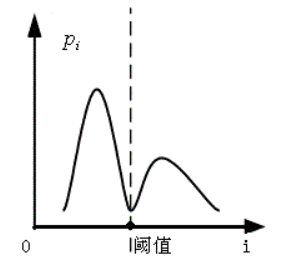图中两个波峰分别为字符笔画和背景部分，波谷为该图像的整体阈值T。目标灰度与背景灰度有明显的差距时采用整体阈值法可以达到很好的二值化效果，但是若输入的图像的目标灰度和背景灰度差距不大或者光照不均匀时，采用 此方法就不能得到较好的效果。

# 3. 平滑去噪

• 数字图像的噪声主要来自于图像的获取阶段。噪声对于图像的预处理非常重要，它会影响图像处理的输入、采集、处理各个环节和识别结果的全过程。特别是图像的输入、采集的噪声是十分关键的问题。如果输入不良伴有较大的噪声,滤波去噪后不能达到理想效果，将必然的严重影响处理的全过程甚至识别结果。噪声去除已经成为图像处理极其重要的步骤，然而现在还没有一个通用的滤波去噪方法对所有的图片适用，一般的图像预处理必须根据实际情况选择不同滤波去噪方法进行比较，最后得出最佳的滤波去噪方法。
• 滤波去噪的方法大体可以分为两大类：频域滤波和空间域滤波。频域滤波一般采用的方法是将空间图像采用快速傅里叶变换转换成频域信息，然后再采用信号处理的滤波方法进行滤波，滤波处理后再通过傅里叶逆变换成空间图像信息，从而达到去噪效果。但是由于频域滤波需要频域转换后再采用滤波器滤波，其实现起来较为复杂，效率也相对较低，而空间域滤波方法速度较快、实现简单、效果很好，因此现在很多研究者中都采用空间域滤波方法对数字图像进行滤波。常用的滤波方法如下:
• 邻域平滑法:利用某- -像素及其邻域像素灰度的平均值作为该中心像素的灰度值，该方法简单但缺点明显，它使图像边界变得模糊。
• 保存边界平滑法:以某一像素作为正方形邻域的中心点，然后选择若干模板，计算每个模板所含像素灰度的方差，计算方差最小的模板所含像素灰度的平均值作为该像素的灰度值。该方法既可以达到去噪的目的，又可以同时保存边界，但计算量庞大。
• 中值滤波算法:中值滤波算法的思想是首先确定-一个以某像素为中心点的邻域，一般为方形邻域，然后将邻域中的各个像素的灰度值进行排序，取其中间值作为中心点像素灰度的新值。这里的邻域- -般可以称为窗口。当窗口在图像中上下左右进行移动后，利用中值滤波算法就可以很好地对图像进行去噪处理，同时使得图像更加平滑。

# 4. 字符分割

• 由于考虑到字符识别的时长及识别准确率，若直接识别一连串字符则效率较低，因此要将输入的一连串字符进行分割，分割成单个的字符再进行识别。
• 现有字符分割的方法可分为三类：
• 第一类是基于统计方法的切分，这种方法适用于字符宽度相差不大的场合。它根据字符的总体统计分布特征，确定字符之间的界线。进行分割判别时以字符的平均字宽作为辅助判别，统计分布特征的代表性和稳定性对切分的正确性及收敛性起很重要的作用;
• 第二类是基于结构的切分，即从字与字之间以及字符本身的结构入手，综合分析、寻找切分的规则:
• 第三类是基于识别的切分方法，即在实际切分前，对各种可能存在的切分结果进行识别，通过对识别结果的判别来选择最终的切分点。

# 5. 字符图像的归一化和细化

## 字符图像的归一化

• 归一化是指将不同大小的字符图像映射到固定大小的字符点阵中来满足识别的需要。归一化可分为线性归一和非线性归一两种方法。
• 线性归一化就是将文字图像按比例线性放大或缩小为同一尺寸，算法简单，失真较小，但是无法调整手写字符的中心位置和笔画密度。
• 非线性归一化强调按照手写字符的特征分布来处理，调整字符的中心位置，削弱那些偏长、偏粗的笔画段，增强一些较短、较细的笔画，或者是突出字符笔画密集的区域，压缩笔画分散的区域。常见的实现方法有:基于字符点密度的方法，基于字符笔画交叉点密度的方法等。

# 字符图像的细化

• 对字符图像细化处理的要求：
• 要保留原有笔画的连续性，不能由于细化造成笔画断开
• 要细化为单线，即笔画宽度只有一个像素
• 细化后的骨架应尽量是原来笔画的中心线
• 要保持文字原有特征，既不要增加，也不要丢失。
• 细化的算法很多，但大多数细化算法都是从字符的边界开始逐层移去黑点，称之为脱壳算法
• 图像的细化算法目前依据是否使用迭代运算可将其分成两类:
• 第一类是非迭代算法，一次即产生骨架，如基于距离变换的方法、游程长度编码细化等。
• 第二类是迭代算法，即重复删除图像边缘满足一定条件的像素，最终得到单像素宽骨架。这类方法依据其检查像素的方法又可再分成串行算法和并行算法。
• 在串行算法中，是否删除像素在每次迭代的执行中是顺序固定的，它不仅仅取决于前次迭代的结果，也取决于本次迭代中已处理过像素的分布情况，
• 在并行算法中，像素的删除与否与像素在图像中的顺序无关，仅取决于前次迭代的结果。
• 常用的有Hilditch细化算法、Deutsch 细化算法、Pavlidis异步细化算法等。 Hilditch细化采用串行处理方式，Deutsch 细化采用并行处理方式，Pavlidis 异步细化则采用并行和串行混合处理方式。

2019-10-15 16:54:17 weixin_44225182 阅读数 522
• ###### MATLAB基础知识大串讲

Matlab基础知识大串讲，具体内容包括matlab下载安装、数据类型、矩阵操作、运算符、字符串处理、数组运算、M文件、变量、控制流、脚本与函数、图形绘制、二维图形绘制、三维图形绘制、四维图形绘制。

33152 人正在学习 去看看 魏伟

## 车牌识别之分离字符

``````Image = imread ('car2.jpg') ;
Image = imresize (Image, [300 450]) ; %调整图像的大小为300*450

gray = 2*Image (:, :, 3) - Image (:, :, 1) - Image (:, :, 2) ;
figure,imshow(gray),title('保留蓝色色域')

l = graythresh (gray) ; %利用Ostu法获取图像的阈值
bw = im2bw (gray, l) ; %根据Ostu法分割车牌图像
figure,imshow(bw),title('二值化后的图像')

bw1 = bwareaopen (bw, 500) ;%删除面积小于500的区域
figure,imshow (bw1) , title ('删除小面积区域后的图像') ;

se = strel ('cube', 15) ;%创建一个边长为15的正方形
bw2 = imclose (bw1, se) ;%闭运算连使整个车牌区域连接在一起
figure,imshow(bw2),title('进行闭运算后的车牌');

stats = regionprops (bw2, 'BoundingBox', 'Centroid') ; %获取车牌矩形区域, 即二值图中值为1的矩形框
bb = stats (1) .BoundingBox;%获取车牌矩形区域左上角坐标及矩形的长和宽, bb (1) 为矩形框左上角的y坐标, bb (2) 为矩形框左上角的x坐标
Img = Image (floor (bb (2) ) :floor (bb (2) +bb (4) ) ,floor (bb (1) ) :floor (bb (1) +bb (3) ) , :) ;
gray = Img (:, :, 2) ;%突出蓝色车牌的白色字体区域
figure,imshow (gray),title('切割图像 提取蓝色色域后')

l = graythresh (gray) ; %利用Ostu法获取阈值
bw3 = im2bw (gray, l) ; %转换为二值图像
figure,imshow (bw3),title('转换为二值图像')

se = strel ('line', 2, 90) ; %创建一个线条形状
bw4 = imclose (bw3, se) ;%闭运算, 连接各字符之间的缝隙
bw5 = imclearborder (bw4) ; %去除与边界相连的部分
bw6 = bwareaopen (bw5, 30) ; %删除面积小于50的区域
figure,imshow (bw6)

stats = regionprops (bw6, 'BoundingBox', 'Centroid') ; %获取各个字的矩形区域坐标
figure,imshow (Img)

for i = 1:length (stats)
bb = stats (i) .BoundingBox;
I = Img (floor (bb (2) ) :floor (bb (2) +bb (4) ) ,floor (bb (1) ) :floor (bb (1) +bb (3) ) , :) ;
str = ['第', num2str(i), '个字'];
figure,imshow (I) ;%展示每个字符
end
``````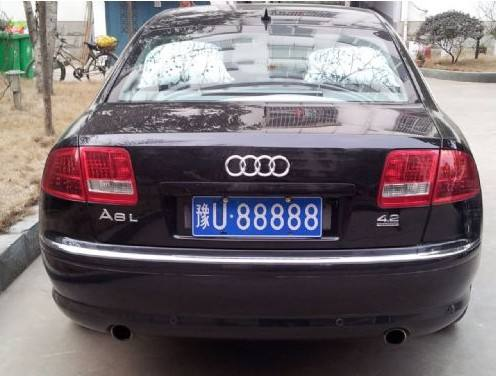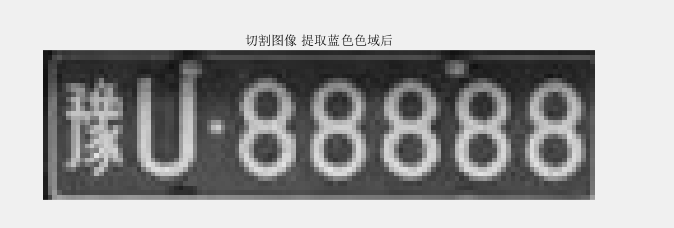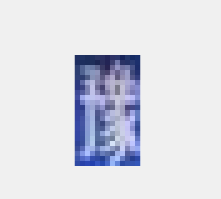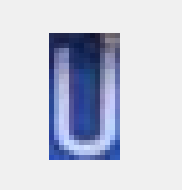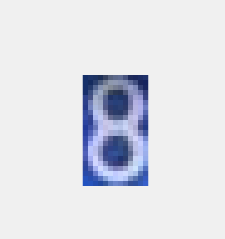2019-11-08 16:38:27 qq_38190041 阅读数 169
• ###### MATLAB基础知识大串讲

Matlab基础知识大串讲，具体内容包括matlab下载安装、数据类型、矩阵操作、运算符、字符串处理、数组运算、M文件、变量、控制流、脚本与函数、图形绘制、二维图形绘制、三维图形绘制、四维图形绘制。

33152 人正在学习 去看看 魏伟

### 一.任务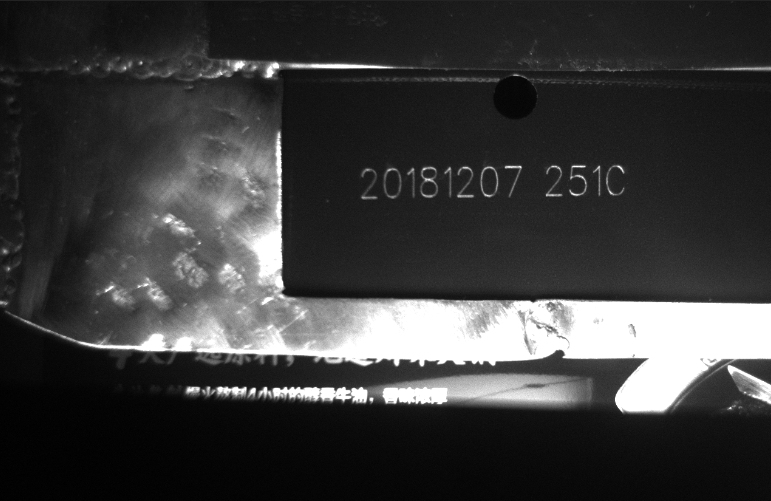Windows10专业版
VS2015企业版
C++ opencv3.2

### 三.图像处理的思路

##### 1.先定位感兴趣区域(字符区域)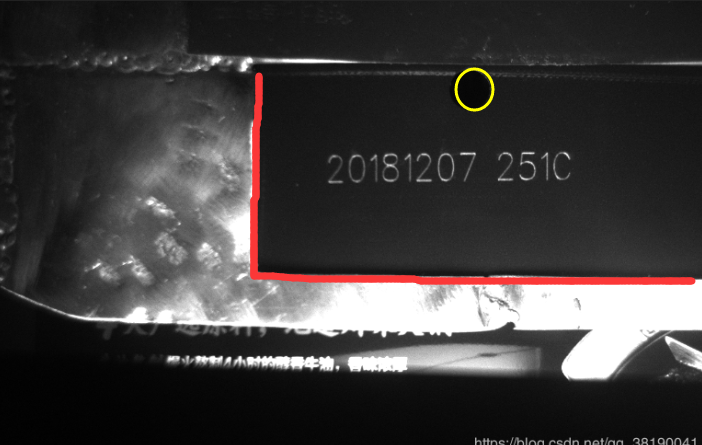##### 2.然后模版匹配(matchTemplate)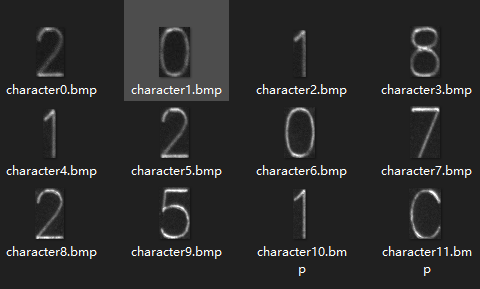### 四.图像处理

1.读入图像
2.转为灰度
3.中值滤波
4.固定阈值二值化。(因为我定位的是圆)
5.霍夫圆检测找到圆心
6.根据圆心剪裁感兴趣区域
7.读取本地文件夹样本
8.进行模版匹配
9.标出模板匹配结果

### 图像：

##### 1.转为灰度中值滤波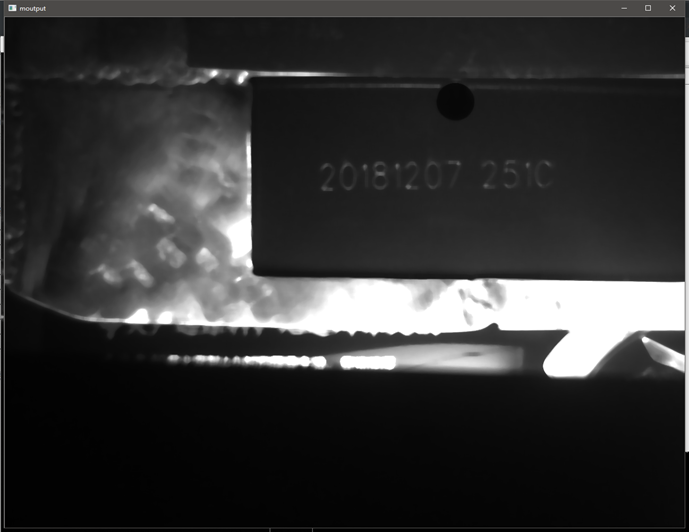##### 2.二值化后霍夫圆检测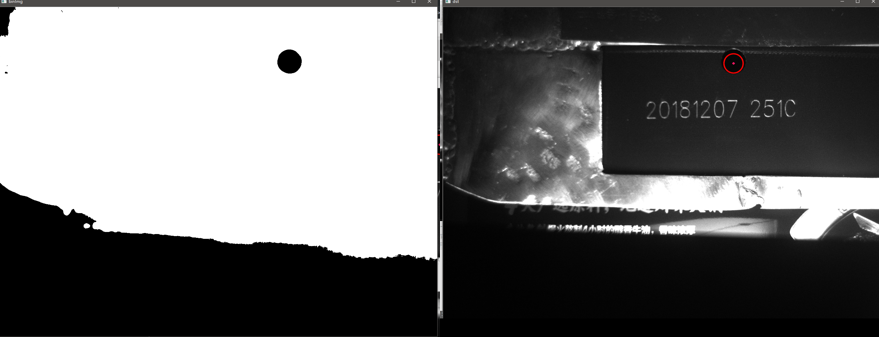##### 3.根据圆心坐标裁出字符区域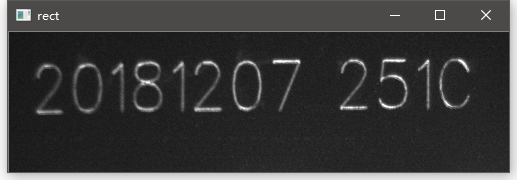##### 3.模版匹配后标记结果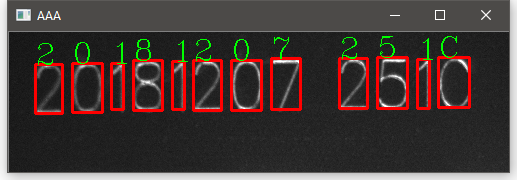``````#include <opencv2/opencv.hpp>
#include <iostream>
#include <math.h>
#include <string>
using namespace cv;
using namespace std;

Mat src, dst, gray, binImg;
Mat eImage;

int main(int argc, char** argv)
{

if (src.empty())
{
return -1;
}
// 窗口命名
namedWindow("test", CV_WINDOW_AUTOSIZE);
imshow("test", src);

//中值滤波
Mat moutput;
medianBlur(src, moutput, 11);
imshow("moutput", moutput);

cvtColor(moutput, gray, CV_BGR2GRAY);

// 固定阈值二值化
threshold(gray, binImg, 15, 255, THRESH_BINARY);
imshow("binImg", binImg);

// 霍夫圆检测
vector<Vec3f> pcircles;
HoughCircles(binImg, pcircles, CV_HOUGH_GRADIENT, 1, 50, 15, 15, 25, 80);
src.copyTo(dst);

// 画圆与圆心
for (size_t i = 0; i < pcircles.size(); i++)
{
Vec3f cc = pcircles[i];
circle(dst, Point(cc, cc), cc, Scalar(0, 0, 255), 2, LINE_AA);
circle(dst, Point(cc, cc), 2, Scalar(98, 23, 255), 2, LINE_AA);
cout << cc << endl;
}
imshow("dst", dst);

// 简陋判断有无圆心坐标
if (pcircles > 0 && pcircles > 0)
{
// 剪裁出字符区域
int start_x = pcircles - 280;
int start_y = pcircles + 80;
int rect_width = 500;
int rect_height = 140;
Rect  rect(start_x, start_y, rect_width, rect_height);
Mat ROI = src(rect);
imshow("rect", ROI);

Mat dst_gray, dst_bin;
cvtColor(ROI, dst_gray, CV_BGR2GRAY);

int height = dst_gray.rows;
int width = dst_gray.cols;

// 取出本地文件的样本
vector<Mat> character_image;
char tempstr = { '2','0','1','8','1','2','0','7','2','5','1','C'};
for (int i = 0; i <= 11; i++)
{
string ImgName = "character";
ImgName = ImgName + to_string(i);
ImgName = "G:\\U_lesson\\图像处理\\实验\\match\\" + ImgName + ".bmp";
character_image.push_back(temp);
}

Mat tmp_dst;
Mat find_dst;
dst_gray.copyTo(find_dst);
cvtColor(find_dst, find_dst, CV_GRAY2BGR);
// 模版匹配
for (int i = 0; i <= 11; i++)
{
Mat temp;
cvtColor(character_image[i],temp, CV_BGR2GRAY);

width = dst_gray.cols - temp.cols + 1;
height = dst_gray.rows - temp.rows + 1;
Mat result(width, height, CV_32FC1);

Point minLoc;
Point maxLoc;
double min, max;
Point temLoc;

dst_gray.copyTo(tmp_dst);
matchTemplate(dst_gray, temp, result, CV_TM_CCORR_NORMED, Mat());

// 找到匹配的最大最小坐标
minMaxLoc(result, &min, &max, &minLoc, &maxLoc, Mat());
temLoc = maxLoc;
cout << temLoc << endl;

// 画出矩形框
rectangle(find_dst, Rect(temLoc.x, temLoc.y, temp.cols, temp.rows), Scalar(0, 0, 255), 2, 8);

string str(1, tempstr[i]);
putText(find_dst, str, Point(temLoc.x, temLoc.y), FONT_HERSHEY_COMPLEX, 1, cv::Scalar(0, 255, 0), 1, 8, 0);

cout << tempstr[i] << endl;
//rectangle(result, Rect(temLoc.x, temLoc.y, temp.cols, temp.rows), Scalar(0, 0, 255), 2, 8);
}

imshow("AAA", find_dst);

}
waitKey(0);
return 0;
}
``````

2015-01-16 10:47:40 u010953266 阅读数 2798
• ###### MATLAB基础知识大串讲

Matlab基础知识大串讲，具体内容包括matlab下载安装、数据类型、矩阵操作、运算符、字符串处理、数组运算、M文件、变量、控制流、脚本与函数、图形绘制、二维图形绘制、三维图形绘制、四维图形绘制。

33152 人正在学习 去看看 魏伟
```%读入图像
bw=rgb2gray(bw);
[m,n]=size(bw);
for i=1:m
forj=1:n
if(bw(i,j)>70)
bw(i,j)=0;
else
%              bw(i,j) =mod( mod(bw(i,j),20),10);
bw(i,j) =mod(bw(i,j),10);
end
end
end;
% imshow(bw);
%输出图像
file=fopen('a.txt','w');
for i=1:m
forj=1:n
if(bw(i,j)>0)
fprintf(file,'%d',bw(i,j));
else
fprintf(file,' ');
end
end
fprintf(file,'\n');
end;
fclose(file);``````bw=imread('./图片/g.jpg');
bw=rgb2gray(bw);
[m,n]=size(bw);
round =10;
for i=1:m
for j=1:n
bw(i,j) = bw(i,j)+round/2-mod(bw(i,j)+round/2,round);
end
end
% for i=1:m
%     for j=1:n
%         if(bw(i,j)>135)
%             bw(i,j)=0;
%         else
% %               bw(i,j) =mod( mod(bw(i,j),20),10);
% %                bw(i,j)=mod( bw(i,j),10);
%               bw(i,j) =mod(bw(i,j)/10,10);
%         end
%     end
% end;
% imshow(bw);

file=fopen('./txt/g.txt','w');
for i=1:m
for j=1:n
fprintf(file,'%c',to_text(bw(i,j)));
%         if(bw(i,j)>0)
%             fprintf(file,'%d',bw(i,j));
%         else
%             fprintf(file,' ');
%         end
end
fprintf(file,'\n');
end;
fclose(file);```

```function [s]  = to_text( g )
charactersARRAY = 'M80V1;:*-. ';
count = size(charactersARRAY);
count=uint8(count(2));
span =uint8(255 / count);
cidx =uint8( g / span);
if cidx > count-1
cidx = count- 1;
end;
s=charactersARRAY(cidx+1);```2013-06-28 13:49:08 diarymaggie 阅读数 125
• ###### MATLAB基础知识大串讲

Matlab基础知识大串讲，具体内容包括matlab下载安装、数据类型、矩阵操作、运算符、字符串处理、数组运算、M文件、变量、控制流、脚本与函数、图形绘制、二维图形绘制、三维图形绘制、四维图形绘制。

33152 人正在学习 去看看 魏伟

```#include "cv.h"
#include "highgui.h"

using namespace cv;

#define PI 3.1415926

static char ch={' ','`','1','2','3','4','5','6','7','8','9','0','-','=','\\',
'q','w','e','r','t','y','u','i','o','p','[',']',
'a','s','d','f','g','h','j','k','l',';','\'',
'z','x','c','v','b','n','m',',','.','/',
'~','!','@','#','\$','%','^','&','*','(',')','_','+','|',
'Q','W','E','R','T','Y','U','I','O','P','{','}',
'A','S','D','F','G','H','J','K','L',':','"',
'Z','X','C','V','B','N','M','<','>','?'};

static int gr={0,7,22,28,31,31,27,32,22,38,32,40, 6,12,20,38,32,26,20,24,40,
29,24,28,38,32,32,26,22,34,24,44,33,32,32,24,16, 6,22,26,22,
26,34,29,35,10, 6,20,14,22,47,42,34,40,10,35,21,22,22,16,14,
26,40,39,29,38,22,28,36,22,36,30,22,22,36,26,36,25,34,38,24,
36,22,12,12,26,30,30,34,39,42,41,18,18,22};

void Image2txt(Mat& mat)
{
int M=mat.rows;
int N=mat.cols;

//将字符按灰度值排序
for(int i=0;i<94;i++)
for(int j=i+1;j<95;j++)
if(gr[i]>gr[j])
{
char tchar=ch[i];
ch[i]=ch[j];
ch[j]=tchar;

int tint=gr[i];
gr[i]=gr[j];
gr[j]=tint;
}

int transWidth=N/8;
int transHeight=M/16;

FILE* fp;
if((fp=fopen("a.txt","w"))==NULL)
exit(0);

for(int i=0;i<transHeight;i++)
{
for(int j=0;j<transWidth;j++)
{
int grayIndex=0;
//计算16*8方块的平均灰度值
for(int i1=0;i1<16;i1++)
{
for(int j1=0;j1<8;j1++)
{
grayIndex+=(int)(255-mat.ptr<uchar>(i*16+i1)[j*8+j1]);
}
}
grayIndex/=(16*8);
grayIndex=gr*grayIndex/255;//拉伸

//寻找字符
int k=0;
while(gr[k+1]<grayIndex)
k++;

fputc(ch[k],fp);
}
fputc(13,fp);
fputc(10,fp);
}
fclose(fp);
}

int main()
{
//读取图像
Mat image;

int M=image.rows;
int N=image.cols;

Image2txt(image);

cvNamedWindow("test",CV_WINDOW_AUTOSIZE);
imshow("test",image);

waitKey(0);
return 0;
}```